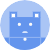search
Search
Publish
Guest 0reps
Thanks for the thanks!
close
account_circle
Profile
exit_to_app
Sign out
search
keyboard_voice
close
Searching Tips
Search for a recipe: "Creating a table in MySQL"
Search for an API documentation: "@append"
Search for code: "!dataframe"
Apply a tag filter: "#python"
Useful Shortcuts
/ to open search panel
Esc to close search panel
to navigate between search results
d to clear all current filters
Enter to expand content previewDoc SearchCode Search BetaSORRY NOTHING FOUND!
mic
Start speaking...Voice search is only supported in Safari and Chrome.
Shrink
Navigate to
A
A
share
thumb_up_alt
bookmark
arrow_backShareTwitterFacebook

# NumPy | zeros method

Programming
chevron_right
Python
chevron_right
NumPy
chevron_right
Documentation
schedule Mar 10, 2022
Last updated
local_offer PythonNumPy
Tags

Numpy's `zeros(~)` method creates a Numpy array with all zeros as its entries.

# Parameters

1. `shape` | `int` or `array-like`

The desired shape of the Numpy array. Providing an `int` would return a one-dimensional flattened array.

2. `dtype`link | `string` or `type` | `optional`

The desired data type for the Numpy array. By default, `dtype=numpy.float64`. If you're sure that the new Numpy array will only contain integers, you should want to specify `dtype=int`.

# Return value

A Numpy array of zeroes, with the shape and type specified by the parameters.

# Examples

## Creating an one-dimensional Numpy array

To create a flattened Numpy array with 3 zeros:

``` np.zeros(3) array([0., 0., 0.]) ```

## Creating a Numpy array of type int

To create a flattened Numpy array with 3 zeroes of type `int`:

``` np.zeros(3, int) array([0, 0, 0]) ```

## Creating a two-dimensional Numpy array

### Using a tuple

To create a 2 by 3 (i.e. 2 rows and 3 columns) matrix filled with zeros using a tuple:

``` np.zeros((2,3)) array([[0., 0., 0.],       [0., 0., 0.]]) ```

### Using an array

To create a 2 by 3 (i.e. 2 rows and 3 columns) matrix filled with zeros using an array:

``` np.zeros([2,3]) array([[0., 0., 0.],       [0., 0., 0.]]) ```

Note that you could also use Numpy arrays as well.